Lee, Y. J., Ko, Y. C., Moon, B. G., and Kim, H. J. (2023), “Surface characterization of paper products by profilometry with a fractal dimension analysis,” BioResources 18(2), 3978-3994.

#### Abstract

A surface profilometry technique was used to characterize the surfaces of paper products. A stylus-contact type profilometer capable of simultaneously generating both surface roughness- and friction-profiles was used. As a stylus for the profilometer, a conical shape whose tip was rounded to have a 0.5 mm curvature radius was designed and successfully employed in both printing & writing (P&W) papers and hygiene papers such as bathroom tissues and kitchen towel. From the profiles, the mean absolute deviation (MAD) from the averages, i.e., R-MAD from the roughness average and F-MAD from the average coefficient of friction, were suggested as the new surface characterization parameters. To elucidate the surface roughness profiles by fractal dimension analysis, the variogram method was applied to get the fractal dimensions of the paper products. Generally, the value of the fractal dimension increased as the surface roughness increased. The surface profilometry technique with the fractal dimension analysis with the variogram method looks promising to gain additional insight on the surface characteristics of paper products.

Surface Characterization of Paper Products by Profilometry with a Fractal Dimension Analysis

Yong Ju Lee,a Young Chan Ko,a Byoung Geun Moon,b and Hyoung Jin Kim a,*

A surface profilometry technique was used to characterize the surfaces of paper products. A stylus-contact type profilometer capable of simultaneously generating both surface roughness- and friction-profiles was used. As a stylus for the profilometer, a conical shape whose tip was rounded to have a 0.5 mm curvature radius was designed and successfully employed in both printing & writing (P&W) papers and hygiene papers such as bathroom tissues and kitchen towel. From the profiles, the mean absolute deviation (MAD) from the averages, i.e., R-MAD from the roughness average and F-MAD from the average coefficient of friction, were suggested as the new surface characterization parameters. To elucidate the surface roughness profiles by fractal dimension analysis, the variogram method was applied to get the fractal dimensions of the paper products. Generally, the value of the fractal dimension increased as the surface roughness increased. The surface profilometry technique with the fractal dimension analysis with the variogram method looks promising to gain additional insight on the surface characteristics of paper products.

DOI: 10.15376/biores.18.2.3978-3994

Keywords: Surface profilometry; Surface roughness profile; Friction profile; Mean absolute deviation; MAD; Fractal dimension analysis; Variogram method

Contact information: a: Department of Forest Products and Biotechnology, Kookmin University, 77 Jeongneung-ro, Seongbuk-gu, Seoul 02707 Republic of Korea; b: Consumer Product Division Packaging Technology Center, Korea Conformity Laboratories, 199, Gasan Digital 1-ro, Geumcheon-gu, Seoul, 08503, Republic of Korea; *Corresponding author: hyjikim@kookmin.ac.kr

INTRODUCTION

Surface characterization plays an important role and finds many applications in the paper industry. Surface roughness and friction are the two main components of surface properties. The former is static and topographical and describes the geometry of the surface (ISO 13565-1 1996; ISO 3274 1996; ISO 4287 1997). The latter is dynamical and mechanical and describes the frictional forces between the two surfaces.

At present, a relationship between the roughness and the friction has not been well established. The reason is that the two properties belong to the extrinsic properties which depend on a test method with its testing conditions (Militky and Bajzik 2001).

Fellers et al. (1998) have reported that the coefficient of friction between the two paper surfaces should be higher for the smoother surfaces because the contact area between the two should be higher. In other words, the friction should be proportional to the contact area, which is inversely proportional to the surface roughness. Meanwhile, other investigators have reported that no relationship between the two should exist, being independent of each other (Garoff et al. 2002; Enomae et al. 2006).

Leach (2010) has demonstrated that even though two surfaces have the same average roughness, the friction can be totally different, supporting the argument that the two should be independent of each other. Thus, it should be prudent to determine both properties for the surface characterization (Ko et al. 2020), in order to avoid the wrong conclusions.

Surface profilometry is a technique to quantify the surface profiles of products. An interest in a stylus-type contact surface profilometric technique is growing in the paper industry because the technique resembles papermaking processes such as creping, coating, printing, lamination, calendaring, and embossing (Park et al. 2021; Moon et al. 2022). It is also similar to the method used for evaluating the quality attributes such as softness, wettability, printability, and absorption (Hollmark 1983a,b; Ampulski et al. 1991; Yokura et al. 2004; Ko et al. 2018, 2020)

As a surface profilometer, the KES-SE surface tester (Kato Tech Company (Kyoto, Japan) has been used to measure either roughness or friction of nonwovens, textiles, and tissue and paper products (Kato Tech 2018a,b). To this end, either a single U-tube stylus or a multiple-wire stylus has been used, although the former has been found to be more discriminating (Yokura et al. 2004). Since their findings, the single U-tube stylus has been preferred by other workers (Ko et al. 2017, 2019). Recently, the method of determining the surface friction of tissue products using the single U-tube stylus has been established as an ISO Standard (ISO 12625-18 2022).

However, it has been realized that this stylus does not work well on high-density paper such as writing & printing papers, kraft paper, and newsprint (Jeong et al. 2019; Moon et al. 2022). For these products, a design of a new stylus has been necessary.

Meanwhile, it was determined that the KES-SE surface tester should have the ability to be used to determine both the roughness and friction-properties simultaneously using the same stylus and under identical operating conditions. This allows one to examine the relationship between the two properties without ambiguity because the two profiles are generated at the exact same positions (Ko et al. 2019, 2020). In this stylus-type contact method, a stylus’ shape and size, its contact force on the sample surface, and its scan speed have been identified as the key variables responsible for generating surface profiles (Kawabata 1980; Jeong et al. 2019; Ko et al. 2020; Moon et al. 2022).

It has been generally accepted that a finer tip size of a stylus should be necessary to determine the surface roughness on a microscale. The finer the tip is, the more accurate it is for roughness measurement. A tip radius of 1.5~2.5 μm (Taylor-Hobson 2002) to 32 μm (TAPPI T575 om-07 2012) has been used for commercial profilometers. Diamond or sapphire has mainly been used to produce a stylus of such a fine tip radius.

Once the surface profiles are obtained, typical parameters such as the roughness average (Ra) and the average coefficient of friction (μ) can be determined. The absolute mean deviation from the average, that is, R-MAD for the roughness, and F-MAD for the friction, have been suggested as new surface parameters (Ko et al. 2020; Park et al. 2021; Moon et al. 2022). F-MAD has been accepted as an ISO Standard for determining the friction properties of tissue products (ISO 12625-18 2022).

Fractal dimension analysis is a technique to quantify the surface profiles (Mandelbrot 1982). Since Mandelbrot introduced the term “fractal geometry,” it has been found that fractal geometry exists everywhere in nature, as well as in the human body (Gleick 1987; Kaye 1989; Briggs 1992; Russ 2013; Barnsley 2014). It seems that fractal geometry should be the rule rather than the exception (Ko et al. 2015). Yet very few studies have been available for applying fractal dimension analysis techniques to quantify the surface profiles of paper products (Ko et al. 2015; Jeong et al. 2019; Moon et al. 2022).

The goal of this work was to characterize paper products by using a stylus-type contact method using a surface profilometer and to examine the relationship between the surface roughness and friction. Another objective was to explore the feasibility of using a fractal dimensional analysis technique to explore the nature of surface profiles.

Surface Roughness Characterization

For the surface roughness characterization using a stylus-contract type profilometer, a surface roughness profile is necessary. Figure 1 shows the surface roughness profiles of a printing and writing (P&W) paper and a bathroom tissue (BT) obtained using the Kato surface tester (Model: KES-SESRU, Kato Tech, Kyoto, Japan) (Kato Tech 2018a).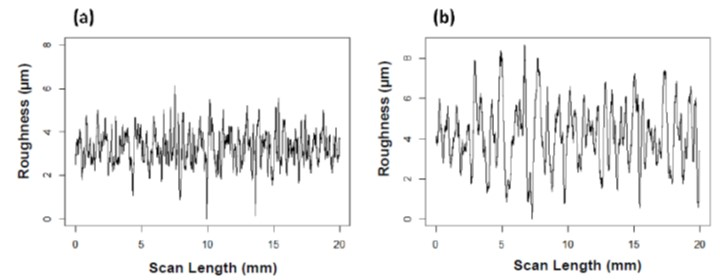Fig. 1. Surface roughness profiles (A: P&W; B: bathroom tissue)

Determination of Surface Roughness Parameters

For a surface roughness profile as shown in Fig. 1, many surface parameters can be determined according to ISO 4287 (1997). The roughness average, Ra, was calculated according to Eq. 1 (Park et al. 2021),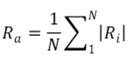(1)

Where Ra is the roughness average (μm), Ri is the roughness (μm) at a scanning point i and N is the number of data points in the scan length. N is calculated from Eq. 2,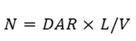(2)

where DAR is the data acquisition rate (Hz or points/s), L is the scan length (mm), and V is the scan speed (mm/s). (Park et al. 2021; Moon et al. 2022).

The spacing distance (SD) between two adjacent points can be calculated from Eq. 3 (Park et al. 2021; Moon et al. 2022).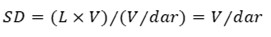(3)

SD may be interpreted as the measuring unit in a roughness profile, indicating a shorter SD with a shorter measuring unit. In Eq. 3, SD should be independent of the size of the stylus, being dependent only on the scan speed (V) and the data acquisition rate (DAR).

As a numerical illustration, at DAR = 1000 Hz, L= 20 mm, and V = 1 mm/s, SD becomes 1 micrometer.

The mean absolute deviation (R-MAD) from Ra has been defined as follows (Park et al. 2021),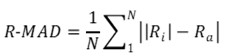(4)

Figure 2 is a graphical representation of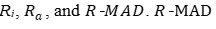is calculated from dividing the shaded area by the scan length and it is shown as the dotted lines. In calculating R-MAD Ra is treated as a constant in the same manner that thickness average is treated as a constant in calculating Ra.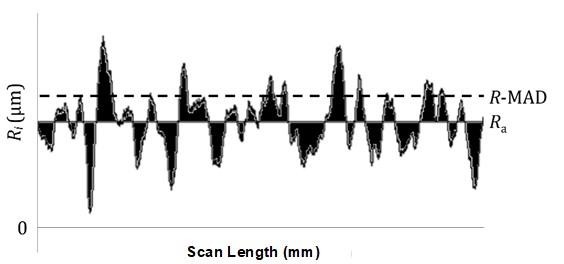Fig. 2. A graphical representation of Ri, Ra, and R-MAD

Friction Characterization

The Kato Surface Tester can generate both surface roughness and friction-profiles at the same time using the same stylus under the same operating conditions. Figure 3 shows the friction profiles of the same samples shown in Fig. 1.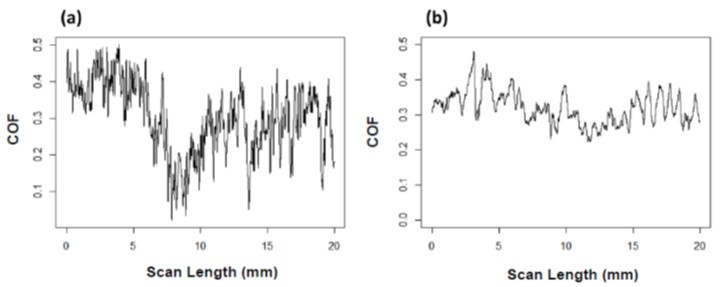Fig. 3. Friction profiles (A: printing and writing; B: bathroom tissue)

The surface friction parameters, average of COF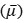and the mean absolute deviation from the average coefficient of friction (F-MAD) are calculated from Eqs. 5 and 6, respectively.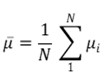(5)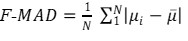(6)

whereis the average of COF, N is number of data points from the scan length, µi is the COF at point i, and F-MAD is the mean absolute deviation from the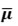(Park et al. 2021; Moon et al. 2022). Here, N is calculated from Eq. 2.

Figure 4 shows a friction profile of the coefficient of friction vs. the scan length. The F-MAD is calculated by dividing the shaded area by the scan length and it is shown as the dotted lines.

In calculating F-MAD, average COF is treated as a constant in similar fashion to the case of R-MAD. F-MAD has been established as the ISO standard method in determining the friction of tissue products (ISO 12625-18 2022).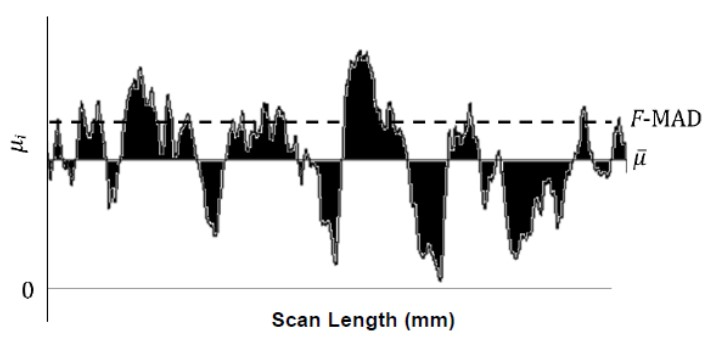Fig. 4. A graphical representation of µi,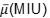and F-MAD

Fractal Geometry Dimension Analysis of Surface Roughness Profiles

In determining the fractal geometry dimension from surface profiles, several methods are available. These include the Richardson Plot or the Box counting method (Richardson 1961; Kuparinen et al. 2005), computer simulation – e.g., Mandelbrot set (Mandelbrot 1981), spectral density analysis with Fast Fourier Transform (PSD/FFT) (Falconer 2004), and the Variogram method (Mandelbrot 1982; Constantine and Hall 1984; Falconer 2004; Hall 1994).

Selecting a fractal dimension analysis method depends on the spacing distance between two adjacent points (Dalton and Herbert 1998; Militky et al. 2001; Ko et al. 2020). It also depends on the type of data acquisition; for example, the box counting method (or the Richardson Methods) has been commonly used to determine the fractal dimension analysis on the data acquired by Image Analyzer. (Kent 1991). In the present paper, the variogram method was used to determine fractal geometry dimensions from the surface profiles (Barnes 2002; Ko et al. 2020).

The Variogram Method

The Variogram method is a quantitative statistical procedure used to characterize the spatial continuity or roughness of a data set using autocorrelation between distances in the surface profile (Barns 2002; Ko et al. 2020). In statistics, autocorrelation means that the observations are dependent on each other. A higher autocorrelation means a higher dependency on each other. A distance between two pints is called lag, so lag K means any two points whose distance is equal to K. Therefore, a larger K means a larger spacing distance between two points (Constantine 1994).

In the variogram method, a variogram is computed from the autocorrelation function as Eq. 7 (Barnes 2002; Ko et al. 2020),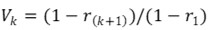(7)

Where Vk is the Variogram at lag k, rk is the autocorrelation at lag k.

Very few papers have been available on the fractal dimension values of paper products whose surface profiles are obtained using a stylus-contact type profilometer (Militky and Bajzik 2001). In the cited work the goal was to determine the fractal dimensions of nonwoven fabric surfaces from the surface roughness profiles obtained from the KES-SE surface tester. To this end, the variogram method by fractal dimension analysis was used. They realized, however, that at least about a couple of thousand surface profile data points would be required. In the present study, 20,000 data points were collected per 20 mm of the sample length, corresponding to a spacing distance of 1 μm.

Calculation of sample autocorrelation from the surface profile data of the surface roughness was performed using the time series analysis in JMP (2021), where a default of lag of K=15. Changing the lag K values used to calculate the autocorrelation may have an effect on the fractal dimension values. Figure 5 shows a plot of the variogram of V_k vs. k of a paper sample (P&W1 in MD) on a log-log scale. It shows that the slope starts to deviate at about log(Lag)= 1.0, corresponding to K=10 in this case. Thus, depending on the samples, the value of K which deviates from the initial slope may be different.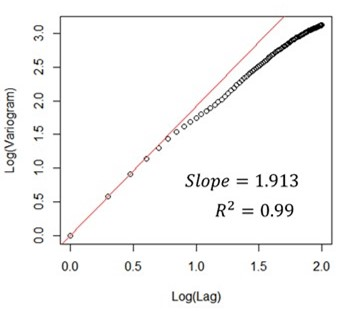Fig. 5. The variogram plot on a log-scale (sample P&W1) in MD

In the variogram method, Fractal Dimension (FD) is determined from Eq. 8,

FD=2-|Slope|/2 (8)

where the slope is determined by linear regression with zero-intercept from the variogram plot in Fig. 5. In Fig. 5, the following regression equation is obtained,

Y = 1.913X, R2=0.99

where Y is log(Variogram), X is log(lag), and M is the slope. In Fig. 5, the following regression equation is obtained,

Y = 1.913X, R2=0.99

Accordingly, the fractal dimension is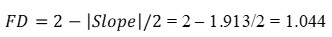According to Eq. 8, when abs(slope) = 2, FD becomes one, indicating that a surface profile should be a straight line. Meanwhile, when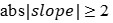, FD becomes zero, or negative, which has no physical meaning. Thus, FD of a roughness profile in one-dimension (e.g, the MD) should lie between 1 and 2 in order to have physical meaning. In this example, the sample surface seems rather smooth because its FD value is close to 1.0.

EXPERIMENTAL

Materials

Three Printing & Writing (P&W) paper specimens, two bathroom tissues (BT), and one paper towel were conditioned for longer than 48 h at a temperature of 23 °C ± 1 °C and a relative humidity (R.H.) of 50% ± 2%, according to ISO standard 187 (ISO 187, 1990). Table 2 shows a list of the samples, as well as their basis weight, thickness, and density.

Table 2. Physical Properties of Samples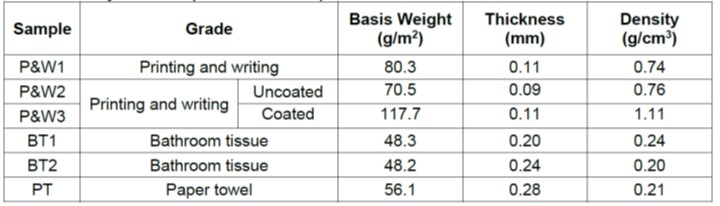The Morphologies of the Samples

SEM (Scanning electron microscopic, JEOL, JSM-7401F, Japan) photographs were taken of these samples. Figure 6 shows the SEM photographs P&W1 and P&W2 at magnifications of 1000x and P&W3 at magnifications of 1000x, and 10000x, respectively.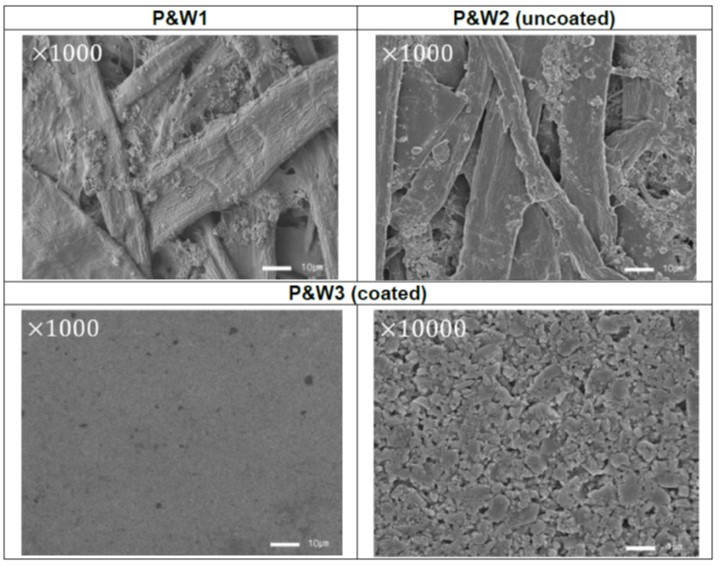Fig. 6. SEM photographs of P&W1, P&W2 (uncoated) and P&W3 (coated) samples

Optical photographs of BT1, BT2, and PT were taken using OSD (OptiTopo surface deviation) equipment (L&W, Sweden). For each sample, three regions having different embossing patterns were taken, as shown in Fig. 7. In the figure, the arrow indicates the machine direction (MD) where scanning was done to get roughness profiles.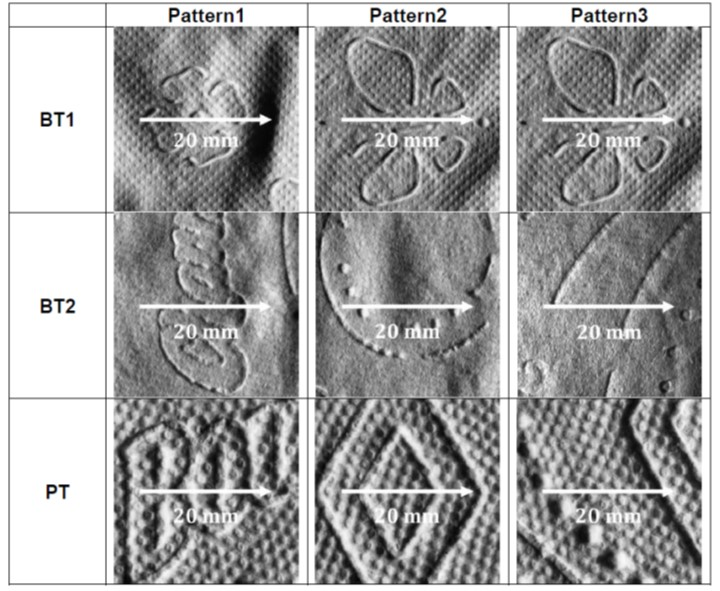Fig. 7. Optical Photographs of Bathroom tissue (BT1 and BT2) and Paper Towel (PT)

The Surface Characterization: Surface Roughness and Friction

The surface tester (KES-SESRU, Kato Tech Co., Ltd., Kyoto, Japan) was used. (Kato Tech 2018a,b; Park et al. 2021).

It is to be noted that in this instrument it is the sample plate, not the stylus, which moves. This is in contrast to a conventional instrument as described in ISO 3274. Thus, the system used in the present work seems very likely to cause less damage to the sample surfaces than the conventional equipment.

Originally, this tester has been set up to employ a single-U type stylus suitable for testing tissue and paper towel products ( Kato Tech 2018a; 2018b; Park et al. 2021; Yokura et al. 2004). Recently, this method has been established as ISO standard to determine the friction of tissue products (ISO 12625-18). It has been, however, realized that this stylus was not suitable for testing P&W samples.

A stylus was designed by rounding the tip of the conical stylus. It was made from a stainless steel (ASTM A681, P21/P20+S). Figure 8 shows the configuration of the profilometer with the tip of the rounded stylus. The contact area between such a stylus design and a sample surface may be assumed to be negligible unless the surface is compressed under the contact force. Thus, the contact area with the sample surface may be safely assumed to be independent of the radius of the curvature of the stylus tip.

The rounded-tip diameter of 1.0 mm was designed and used for both P&W samples and tissue samples.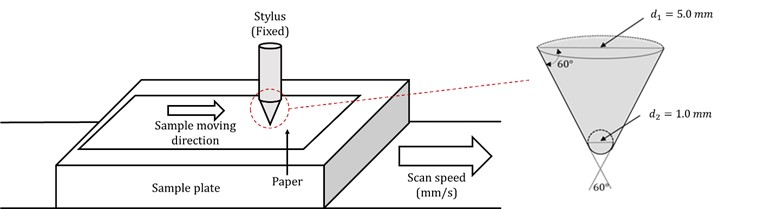Fig. 8. Configuration of the profilometer with the stylus

The testing conditions were as follows: scan length 20 mm; scan speed 1 mm/s; and data acquisition rate 1000 Hz (or 1000 point/s). For each sample, 10 measurements were taken in the machine direction (MD) The testing was performed at 23 °C ± 1 °C and at R.H. of 50% ± 2%.

RESULTS AND DISCUSSION

Determination of Surface Roughness Parameters

Printing & Writing(P&W) Samples

Figure 9 shows the roughness profiles of P&W samples in the machine direction(MD). It shows that the profiles were widely different from each other.

The roughness average (Ra) and the mean absolute deviation (R-MAD) were calculated using Eqs. 1 and 4, respectively. The coefficient of variation (COV) in percentage was determined from 10 measurements for each sample.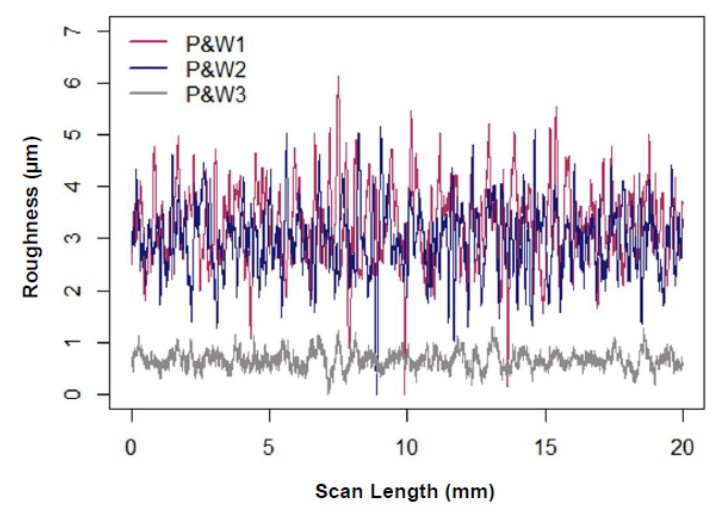Fig. 9. Roughness profiles of P&W Samples

Table 3 shows the results. A couple of items are noted in the table. First, R-MAD and its COV were significantly lower than its corresponding Ra and COV. This can support the argument what R-MAD should be a measure of the true surface profile, unlike Ra which depends on the testing conditions, as described in Fig. 2. It shows that Ra is treated as a constant in calculating R-MAD.

Second, comparing P&W2 (uncoated) with its coated P&W3 indicates that both Ra and R-MAD had been reduced remarkably by about 70%. Similar results have been obtained by other investigators (Jeong et al. 2019; Park et al. 2021). This confirms that the effect of coating on a paper surface can be determined by determining the Ra and R-MAD from the roughness profiles using a surface profilometer.

Table 3. Results for Ra and R-MAD of P&W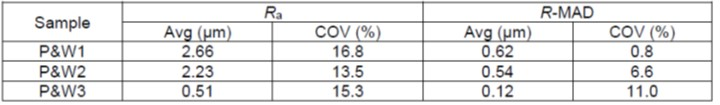* Machine direction

Bathroom Tissue (BT1, BT2) and Paper Towel (PT) Samples

Table 4 shows the results of Ra and R-MAD of Tissue (BT1, BT2) and Towel (PT) samples. Figure 10 shows the roughness profiles of Bathroom tissues (BT1 and BT2) and paper towel (PT) according to their embossing patterns, respectively.

The figure shows that Ra and R-MAD had been affected by the embossing patterns of the samples. As shown in Fig. 7 for the BT1 sample, Pattern 2 was much more remarkable than its Pattern 3, resulting in a high value of Ra and R-MAD. Nevertheless, the difference in R-MAD between the two was much smaller than that of Ra, i.e., 4% vs. 56%. This indicates that an embossing pattern should influence R-MAD much less than Ra.

Table 4. Results for Ra and R-MAD of BT1, BT2, and PT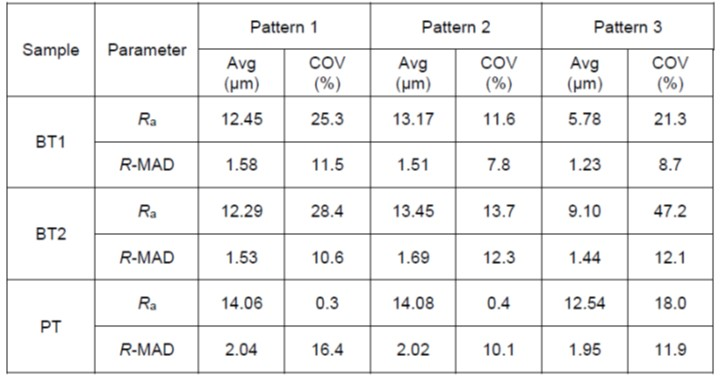* Machine direction

Most commercial tissues and paper towels are embossed. Therefore, it is extremely challenging to determine the Ra of such products. To avoid this problem, Hollmark (1983a,b) tried to avoid the embossing regions for determining the surface roughness. A determination of R-MAD should eliminate the problem, as shown in the present study.

The reason why R-MAD is less sensitive to embossing may be explained from Eq. 2 and Fig. 2, which show that in calculating R-MAD, Ra is treated as a constant. Accordingly, while Ra depends on testing conditions as well as the embossing pattern of a sample, R-MAD should be much less dependent on them.

Determination of Friction Parameters

Printing & Writing(P&W) Samples

Figure 10 shows the friction profiles of P&W samples. Table 5 shows the values of average coefficient of friction () and F-MAD of the P&W samples. It shows that F-MAD was much lower than, as is the case with Ra and R-MAD shown in Table 3. As shown in eq. 6, in calculating F-MAD, is treated as a constant. Thus, while depends on the testing conditions, F-MAD should be much less dependent on them.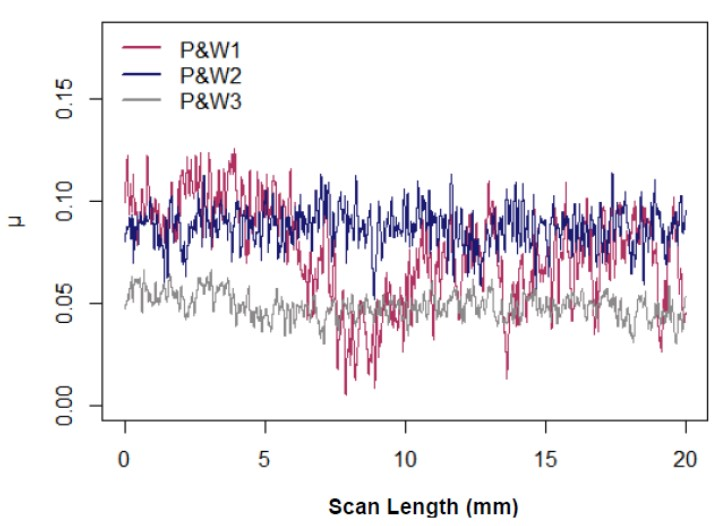Fig. 10. Friction profiles of P&W samples

The effect of coating onand F-MAD can be seen by comparing the values ofand F-MAD of P2 (uncoated) with those of P3 (coated). Table 6 shows bothand F-MAD of P2 decreased after the coating (P3), which is consistent with the roughness as discussed earlier. However, the degree of the decrease in F-MAD was much more significant than in(60% for F-MAD vs. 28 % for). This suggests that the effect of coating can be better determined from F-MAD than.

Table 5. Results forand F-MAD of P&W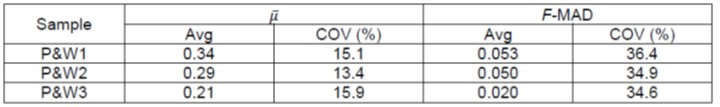* Machine direction

Table 6. Effects of Coating on Friction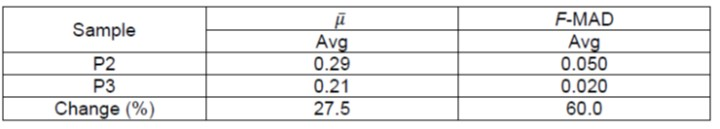Bathroom Tissue (BT1, BT2) and Paper Towel(PT) Samples

Table 7 shows the results ofand F-MAD of the tissue and towel samples.

Table 7. Results forand F-MAD of BT1, BT2, and PT